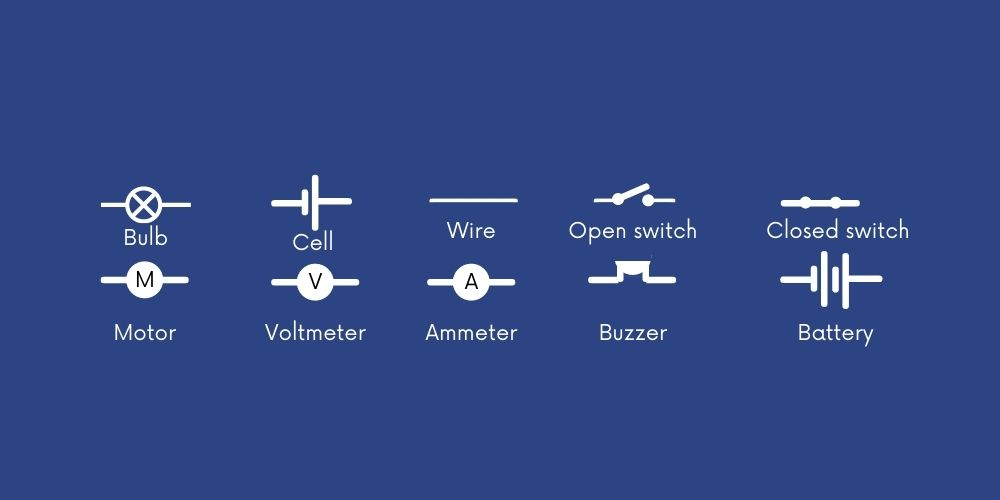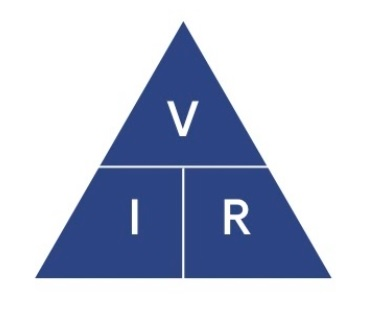Select Page

## Have a read of this blog and we take a look at the three key areas we think you need to cover in your learning and test prep.

### Circuit diagrams

You need to be able to recognise the difference between a series circuit and a parallel circuit. At KS3 you will hopefully experience building these circuits in your science lessons and this is one of the best ways to learn. As you build them you will gain a better understanding of why they work and what happens if you alter the pathway for the electrical current to flow. This is important as you will be expected to be able to explain this.

In a series circuit the current flows in one complete loop, all components are on the same path and the current in a series circuit is the same at any point. In a parallel circuit, there are branches for the current to choose different pathways to flow. In both types of circuits, the circuit or chosen pathway must be complete for the current to flow.

### Circuit symbols

When drawing circuits and their symbols, it is always best to draw them with a sharp pencil and a ruler, this makes it clear which components you are drawing. There are many symbols you need to learn, recognise and potentially be able to draw. In an assessment you could be given a circuit to label or you could be given a list of apparatus and told to draw the correct diagram. There are many different circuit symbols you need to learn, we recommend you make sure you can draw the following from memory:### Calculating current, resistance and potential difference

In KS3 Physics there are some important equations you need to learn and they will be needed for your KS4 Physics as well. One of these is the equation used to calculate Current:The formula triangle above is one you need to learn:

Current (I) = Potential Difference (V) / Resistance (Ω)

It is important you are able to rearrange and write it out in any of the 3 possibilities, as you may need to use it in different ways depending on the question. Make sure you practise writing these regularly so you can recall them in any format required.

### Remember:

💡   Differences between series and parallel circuits
💡   How to draw the different components in a circuit
💡   Learn the equations that connect current, resistance and potential difference